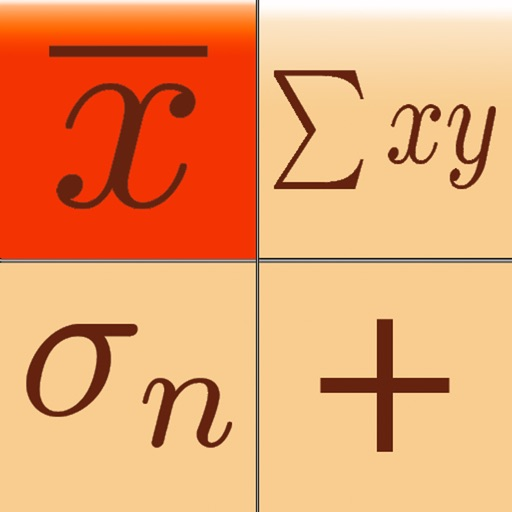## Statistics Calculator+++ is a scientific calculator with statistical, regression analysis, probability distributions, confidence intervals, and hypothesis tests capabilities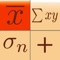# Statistics Calculator+++

by Dogahe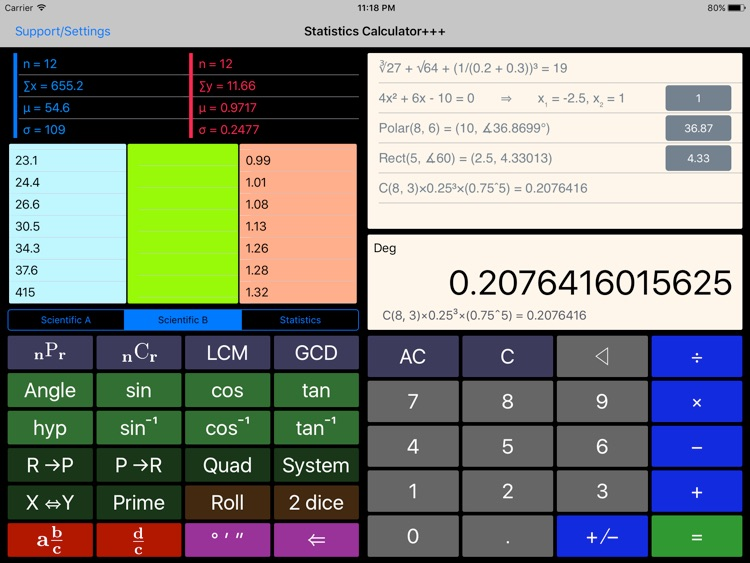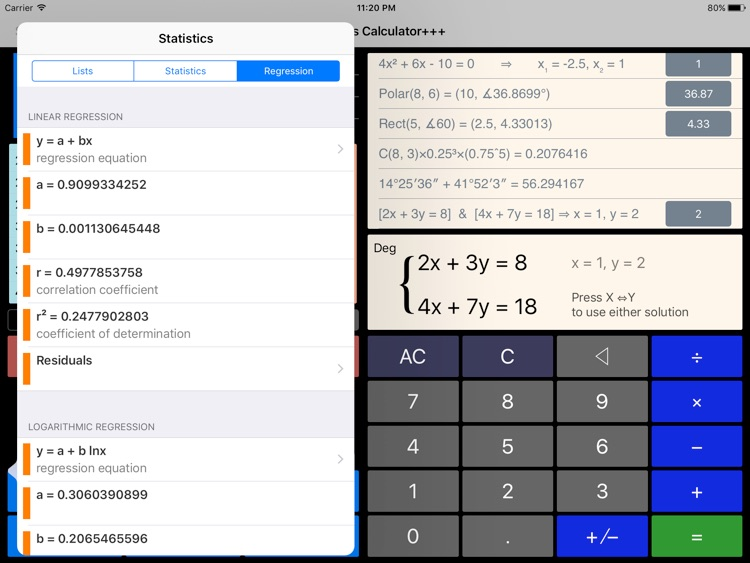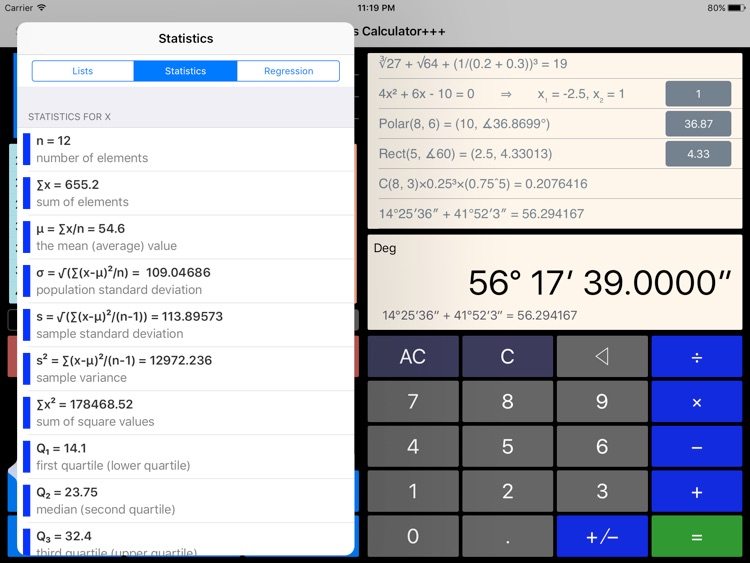Statistics Calculator+++ is a scientific calculator with statistical, regression analysis, probability distributions, confidence intervals, and hypothesis tests capabilities.### App Details

Version
1.13
Rating
(28)
Size
4Mb
Genre
Utilities Finance
Last updated
November 18, 2020
Release date
November 21, 2012

• \$0.99

### App Screenshots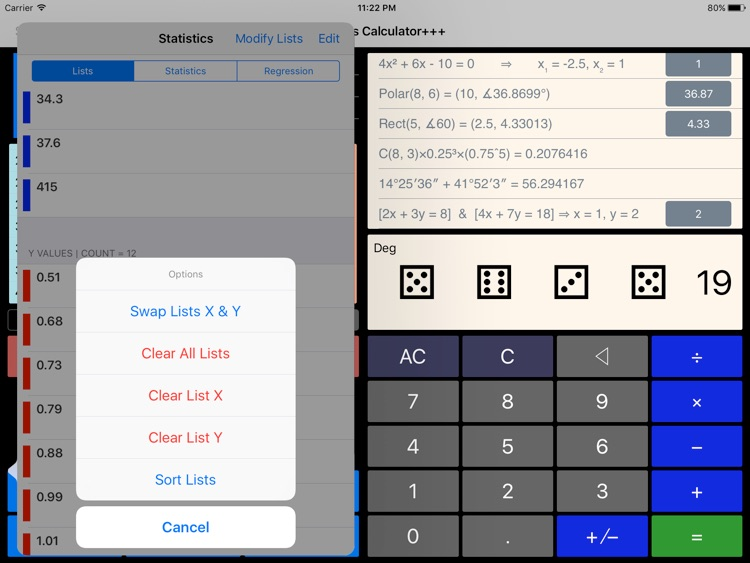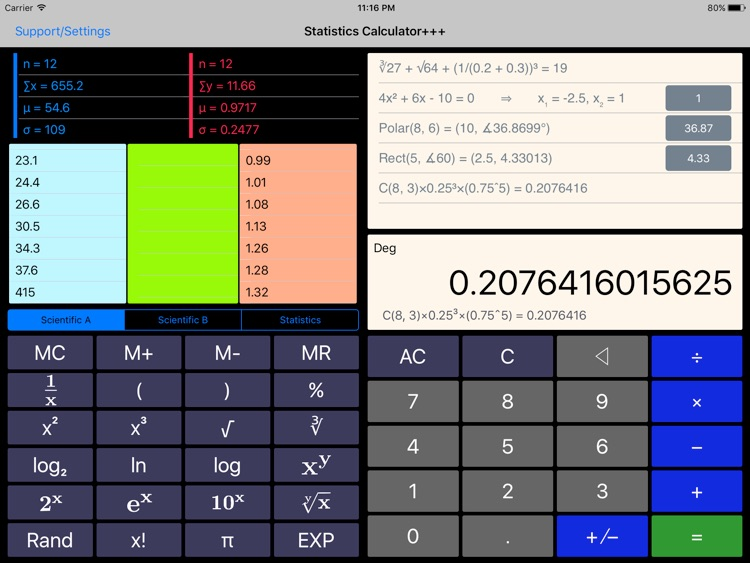### App Store Description

Statistics Calculator+++ is a scientific calculator with statistical, regression analysis, probability distributions, confidence intervals, and hypothesis tests capabilities.

In designing the statistics functions of this app we had one thing in mind: The user should not need to read a single line of manual to perform the statistical analysis.

Simply use Add X, Add Y, and Add f buttons to enter the elements of the lists and frequencies, if any, and you are done. All the statistics and regression models are only a few taps and swipes away. Tap the List button to see the lists entered. The elements of the lists are interpreted independently (as two separate lists) and jointly for regression analysis (if the size of the lists are equal). You can also take advantage of a new import feature to bring in your data from Microsoft Excel or Apple Numbers by first converting them into .csv format.

The sum, mean, population standard deviation, sample standard deviation, sample variance, sum of squares, min, max, quartiles, and sum of multiplications of two lists are presented in a table. You can see the box and whiskers plot and histogram of the data.

For regression analysis linear, logarithmic, exponential, power, and quadratic regressions are calculated. Statistics Calculator+++ has an extremely simple and intuitive user interface to choose the regression model and calculate predictions for x and y values and view residuals and residual sum of squares. It also graphs the regression model and how it fits around the data entered.

You have the capability to save multiple lists of data and use any of them as list X, list Y, or list f. Access Lists Editor feature by tapping the icon on top right of the Statistics Lists view. Furthermore, all these lists persist in the memory. Therefore, if you close and the relaunch the app the lists are there for you.

Normal distribution functionality of TI-83/TI-84 (normalpdf, normalcdf, invNorm, ShadeNorm), Student's t-distribution (tpdf, tcdf, invT), Chi-Squared distribution, F distribution, Binomial distribution (binompdf, binomcdf), Poisson distribution (poissonpdf, poissoncdf), and Geometric distribution (geometpdf, geometcdf) are all possible with this app with an easy to use, and beautiful user interface. In addition you have Beta and Gamma Distributions.

Confidence interval calculation and step by step explanation for "One Population Proportion", "Difference in Two Population Proportions", "Population Mean", and "Difference in Population Means".

Hypothesis test for "Population Proportion", "Difference in Two Population Proportions", "Population Mean" and "Population Mean Difference" with known and unknown population standard deviations.

Other major features of Statistics Calculator+++ are:
- The left pane scrolls to reveal more functionality without a need to switch modes.
- You see the expression as you enter and it keeps a history of your calculations
- Capability of performing fraction manipulations and converting between different formats (proper, improper, and mixed fractions) and seamless integration of fraction, integer, and decimal manipulations.
- Capability of inputting degrees, minutes, and seconds for angles and converting between degrees-minutes-seconds format and decimal format.
- Capability of calculating permutation, combination, Least Common Multiple (LCM), Greatest Common Divisor (GCD), and prime factorization.
- Quadratic and system of two linear equations solver
- Rectangular to polar and polar to rectangular coordinate conversion (extremely useful for phasor calculations for electrical engineers)
- Throws a set of dice (choice of 1 die to 4 dice)
- Backspace to delete last digit entered.

This is an ongoing project and we are working on more features and functions to be added to every update of the app.

You are going to love the simplicity of the user interface and yet have the powerful functionality with which Statistics Calculator+++ is loaded.

Disclaimer:
AppAdvice does not own this application and only provides images and links contained in the iTunes Search API, to help our users find the best apps to download. If you are the developer of this app and would like your information removed, please send a request to [email protected] and your information will be removed.